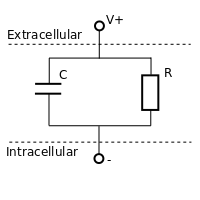# Leaky Integrate and Fire Neuron Discussion and Code

Git repository: https://bitbucket.org/erikjohnson24/eriksresearchcode

The Leaky Integrate and Fire (LIF) neuron is one of the most basic models of neural excitability and spiking. The LIF neuron is based on simplified electrical modeling of the semi-permeable membrane and lipid bilayer that is so fundamental to cells. Due to the selective ion channels embedded in the membrane, the cell maintains differing concentrations of charged ions in the extracellular and intracellular medium. This imbalance of ions results in a measurable membrane voltage, usually negative and in the 10s of millivolts.

Measurements and mathematical models of this voltage date back at least to Louis Lapicque, who studied its integrative properties. The integrative properties can be thought of as a capacitor circuit. Brunel and vanRossum have a nice historical discussion in “Lapicque’s 1907 paper: from frogs to integrate-and-fire”, which is well worth a read.

This was later extended to a resistor-capacitor circuit, known as an RC circuit. In the RC circuit, the capacitor provides an integrative component, building up voltage over time in response to a current input. The resistor, on the other hand, provides the ‘leak’ term, which limits the build up of voltage. In the signal processing world, this is a first-order low-pass analog filter. Ionic currents serve as the input to this filter and the output is the measured voltage.Simple diagram of the RC circuit

This can be represented mathematically as$\tau \frac{dv}{dt}=R I(t)- (V(t)-V_{\text{rest}})$

where$\tau$ is the time-constant and is equal to$RC$, with units of seconds.$V_{\text{rest}}$ is the resting membrane potential of the cell, typically somewhere around -50 millivolts.

In this model, a positive step current will result in an exponential rise in voltage to a fixed level, and an exponential decay back to$V_{\text{rest}}$ when the step is finished.

The final piece needed to make this a spiking neuron model is to incorporate a threshold,$\theta$. When$V(t)=\theta$

a spike is fired and the voltage reset to$V_{\text{rest}}$.

I’ve uploaded my matlab implementation of the LIF neuron to https://bitbucket.org/erikjohnson24/eriksresearchcode/src/master/neural/lif.m

This is specified as

function [voltage, spikes]=lif(current,threshold,tau,R,fs)

Where voltage is$V(t)$ and spikes is an array of the spike times. Current is the input current waveform, threshold is the threshold in millivolts. The variable$\tau$ is the time constant in seconds and$R$ the resistance in ohms. Finally, fs is the sampling frequency of the simulation.

Example usage is given in test_lif.m

Although the LIF is a simple model to analyze and explain, it fails to explain much observed neural activity. The predicted voltage is only loosely related to membrane voltages recorded experimentally (there is some relation to the subthreshold voltage, but that’s about it). The LIF is just the tip of the iceberg in neural modeling, but it provides a good starting place.

Posted on